# Simple Parameterization and Trivial Plans — Part 2

The SQLPerformance.com bi-weekly newsletter keeps you up to speed on the most recent blog posts and forum discussions in the SQL Server community.

eNews is a bi-monthly newsletter with fun information about SentryOne, tips to help improve your productivity, and much more.

Subscribe

Featured AuthorItzik is a T-SQL trainer, a co-founder of SolidQ, and blogs about T-SQL fundamentals and query tuning.

Itzik’s Posts

[ This series:  Part 1 | Part 2 | Part 3 | Part 4 | Part 5 | Part 6 ]

# Parameter Data Types

As mentioned in the first part of this series, one of the reasons it is better to explicitly parameterize is so you have full control over parameter data types. Simple parameterization has a number of quirks in this area, which can result in more parameterized plans being cached than expected, or finding different results compared with the unparameterized version.

When SQL Server applies simple parameterization to an ad-hoc statement, it makes a guess about the data type of the replacement parameter. I’ll cover the reasons for the guessing later in the series.

For the time being, let’s look at some examples using the Stack Overflow 2010 database on SQL Server 2019 CU 14. Database compatibility is set to 150, and the cost threshold for parallelism is set to 50 to avoid parallelism for now:

```ALTER DATABASE SCOPED CONFIGURATION
CLEAR PROCEDURE_CACHE;
GO
SELECT U.DisplayName
FROM dbo.Users AS U
WHERE U.Reputation = 252;
GO
SELECT U.DisplayName
FROM dbo.Users AS U
WHERE U.Reputation = 25221;
GO
SELECT U.DisplayName
FROM dbo.Users AS U
WHERE U.Reputation = 252552;
```

These statements result in six cached plans, three Adhoc and three Prepared: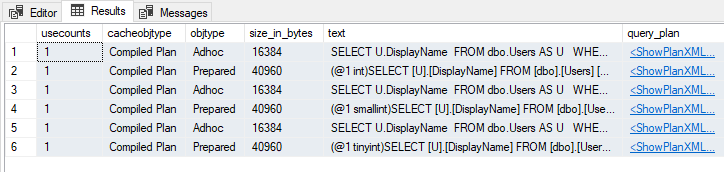Different guessed types

Notice the different parameter data types in the Prepared plans.

## Data Type Inference

The details of how each data type is guessed are complex and incompletely documented. As a starting point, SQL Server infers a basic type from the textual representation of the value, then uses the smallest compatible subtype.

For a string of numbers without quotation marks or a decimal point, SQL Server chooses from `tinyint`, `smallint`, and `integer`. For such numbers beyond the range of an `integer`, SQL Server uses `numeric` with the smallest possible precision. For example, the number 2,147,483,648 is typed as `numeric(10,0)`. The `bigint` type isn’t used for server-side parameterization. This paragraph explains the data types selected in the prior examples.

Strings of numbers with a decimal point are interpreted as `numeric`, with a precision and scale just large enough to contain the value provided. Strings prefixed with a currency symbol are interpreted as `money`. Strings in scientific notation translate to `float`. The `smallmoney` and `real` types aren’t employed.

The `datetime` and `uniqueidentifer` types cannot be inferred from natural string formats. To get a `datetime` or `uniqueidentifier` parameter type, the literal value must be provided in ODBC escape format. For example `{d '1901-01-01'}`, `{ts '1900-01-01 12:34:56.790'}`, or `{guid 'F85C72AB-15F7-49E9-A949-273C55A6C393'}`. Otherwise, the intended date or UUID literal is typed as a string. Date and time types other than `datetime` aren’t used.

General string and binary literals are typed as `varchar(8000)`, `nvarchar(4000)`, or `varbinary(8000)` as appropriate, unless the literal exceeds 8000 bytes in which case the `max` variant is used. This scheme helps avoid the cache pollution and low level of reuse that would result from using specific lengths.

It isn’t possible to use `CAST` or `CONVERT` to set the data type for parameters for reasons I’ll detail later in this series. There is an example of this in the next section.

I won’t cover forced parameterization in this series, but I do want to mention the rules for data type inference in that case have some important differences compared to simple parameterization. Forced parameterization wasn’t added until SQL Server 2005, so Microsoft had the opportunity to incorporate some lessons from the simple parameterization experience, and didn’t have to worry much about backward-compatibility issues.

## Numeric Types

For numbers with a decimal point and whole numbers beyond the range of `integer` , the inferred type rules present special problems for plan reuse and cache pollution.

Consider the following query using decimals:

```ALTER DATABASE SCOPED CONFIGURATION
CLEAR PROCEDURE_CACHE;
GO
DROP TABLE IF EXISTS dbo.Test;
GO
CREATE TABLE dbo.Test
(
SomeValue decimal(19,8) NOT NULL
);
GO
SELECT
T.SomeValue
FROM dbo.Test AS T
WHERE
T.SomeValue >= 987.65432
AND T.SomeValue < 123456.789;
```

This query qualifies for simple parameterization. SQL Server chooses the smallest precision and scale for the parameters able to contain the supplied values. This means it chooses `numeric(8,5)` for `987.65432` and `numeric(9,3)` for `123456.789`: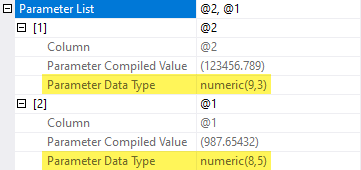Inferred numeric data types

These inferred types don’t match the `decimal(19,8)` type of the column, so a conversion around the parameter appears in the execution plan: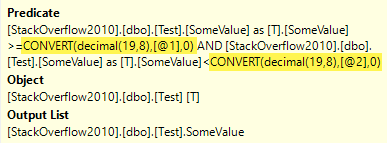Conversion to column type

These conversions only represent a small runtime inefficiency in this particular case. In other situations, a mismatch between the column data type and the inferred type of a parameter might prevent an index seek or require SQL Server to do extra work to manufacture a dynamic seek.

Even where the resulting execution plan seems reasonable, a type mismatch can easily affect plan quality due to the effect of the type mismatch on cardinality estimation. It’s always best to use matching data types, and to pay careful attention to the derived types resulting from expressions.

### Plan Reuse

The main issue with the current plan is the specific inferred types affecting cached plan matching and therefore reuse. Let’s run a couple more queries of the same general form:

```SELECT
T.SomeValue
FROM dbo.Test AS T
WHERE
T.SomeValue >= 98.76
AND T.SomeValue < 123.4567;
GO
SELECT
T.SomeValue
FROM dbo.Test AS T
WHERE
T.SomeValue >= 1.2
AND T.SomeValue < 1234.56789;
GO
```

Now look at the plan cache:

```SELECT
CP.usecounts,
CP.objtype,
ST.[text]
FROM sys.dm_exec_cached_plans AS CP
CROSS APPLY sys.dm_exec_sql_text (CP.plan_handle) AS ST
WHERE
ST.[text] NOT LIKE '%dm_exec_cached_plans%'
AND ST.[text] LIKE '%SomeValue%Test%'
ORDER BY
CP.objtype ASC;
```

It shows an AdHoc and Prepared statement for each query we submitted: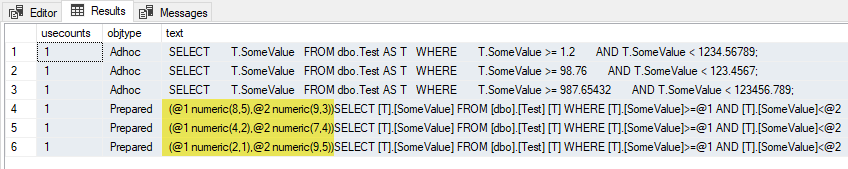Separate prepared statements

The parameterized text is the same, but the parameter data types are different, so separate plans are cached, and no plan reuse occurs.

If we continue to submit queries with different combinations of scale or precision, a new Prepared plan will be created and cached each time. Remember the inferred type of each parameter isn’t limited by the column data type, so we could end up with a tremendous number of cached plans, depending on the numeric literals submitted. The number of combinations from `numeric(1,0)` to `numeric(38,38)` is already large before we think about multiple parameters.

### Explicit Parameterization

This problem doesn’t arise when we use explicit parameterization, ideally choosing the same data type as the column the parameter is compared with:

```ALTER DATABASE SCOPED CONFIGURATION
CLEAR PROCEDURE_CACHE;
GO
DECLARE
@stmt nvarchar(4000) =
N'SELECT T.SomeValue FROM dbo.Test AS T WHERE T.SomeValue >= @P1 AND T.SomeValue < @P2;',
@params nvarchar(4000) =
N'@P1 numeric(19,8), @P2 numeric(19,8)';

EXECUTE sys.sp_executesql
@stmt,
@params,
@P1 = 987.65432,
@P2 = 123456.789;

EXECUTE sys.sp_executesql
@stmt,
@params,
@P1 = 98.76,
@P2 = 123.4567;

EXECUTE sys.sp_executesql
@stmt,
@params,
@P1 = 1.2,
@P2 = 1234.56789;
```

With explicit parameterization, the plan cache query shows only one plan cached, used three times, and no type conversions needed: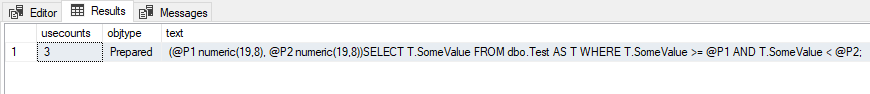Explicit parameterization

As a final side note, I’ve used `decimal` and `numeric` interchangeably in this section. They are technically different types, though documented to be synonyms and behaving equivalently. This is usually the case, but not always:

```-- Raises error 8120:
-- Column 'dbo.Test.SomeValue' is invalid in the select list
-- because it is not contained in either an aggregate function
-- or the GROUP BY clause.
SELECT CONVERT(decimal(19,8), T.SomeValue)
FROM dbo.Test AS T
GROUP BY CONVERT(numeric(19,8), T.SomeValue);
```

It’s probably a small parser bug, but it still pays to be consistent (unless you’re writing an article and want to point out an interesting exception).

## Arithmetic Operators

There’s one other edge case I want to address, based on an example given in the documentation, but in a bit more detail (and perhaps accuracy):

```-- The dbo.LinkTypes table contains two rows

-- Uses simple parameterization
SELECT r = CONVERT(float, 1./ 7)

-- No simple parameterization due to
-- constant-constant comparison
SELECT r = CONVERT(float, 1./ 7)
WHERE 1 = 1;
```

The results are different, as documented: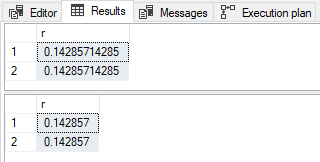Different results

### With Simple Parameterization

When simple parameterization occurs, SQL Server parameterizes both literal values. The `1.` value is typed as `numeric(1,0)` as expected. Somewhat inconsistently, the `7` is typed as `integer` (not `tinyint`). The rules of type inference have been built over time, by different teams. Behaviours are maintained to avoid breaking legacy code.

The next step involves the `/` arithmetic operator. SQL Server requires compatible types before performing the division. Given `numeric` (`decimal`) has a higher data type precedence than `integer`, the `integer` will be converted to `numeric`.

SQL Server needs to implicitly convert the `integer` to `numeric`. But which precision and scale to use? The answer could be based on the original literal, as SQL Server does in other circumstances, but it always uses `numeric(10)` here.

The data type of the result of dividing a `numeric(1,0)`by a `numeric(10,0)` is determined by another set of rules, given in the documentation for precision, scale, and length. Plugging the numbers into the formulas for result precision and scale given there, we have:

• Result precision:
• p1 - s1 + s2 + max(6, s1 + p2 + 1)
• = 1 - 0 + 0 + max(6, 0 + 10 + 1)
• = 1 + max(6, 11)
• = 1 + 11
• = 12
• Result scale:
• max(6, s1 + p2 + 1)
• = max(6, 0 + 10 + 1)
• = max(6, 11)
• = 11

The data type of `1. / 7` is, therefore, `numeric(12, 11)`. This value is then converted to `float` as requested and displayed as `0.14285714285` (with 11 digits after the decimal point).

### Without Simple Parameterization

When simple parameterization isn’t performed, the `1.` literal is typed as `numeric(1,0)` as before. The `7` is initially typed as `integer` also as seen previously. The key difference is the `integer` is converted to `numeric(1,0)`, so the division operator has common types to work with. This is the smallest precision and scale able to contain the value `7`. Remember simple parameterization used `numeric(10,0)` here.

The precision and scale formulas for dividing `numeric(1,0)` by `numeric(1,0)` give a result data type of `numeric(7,6)`:

• Result precision:
• p1 - s1 + s2 + max(6, s1 + p2 + 1)
• = 1 - 0 + 0 + max(6, 0 + 1 + 1)
• = 1 + max(6, 2)
• = 1 + 6
• = 7
• Result scale:
• max(6, s1 + p2 + 1)
• = max(6, 0 + 1 + 1)
• = max(6, 2)
• = 6

After the final conversion to `float`, the displayed result is `0.142857` (with six digits after the decimal point).

The observed difference in the results is therefore due to interim type derivation (`numeric(12,11)` vs. `numeric(7,6)`) rather than the final conversion to `float`.

If you need further evidence the conversion to `float` isn’t responsible, consider:

```-- Simple parameterization
SELECT r = CONVERT(decimal(13,12), 1. / 7)

-- No simple parameterization
SELECT r = CONVERT(decimal(13,12), 1. / 7)
OPTION (MAXDOP 1);
```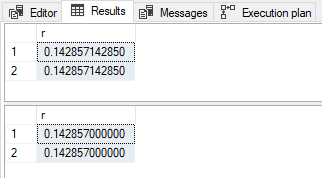Result with decimal

The results differ in value and scale as before.

This section doesn’t cover every quirk of data type inference and conversion with simple parameterization by any means. As said before, you’re better off using explicit parameters with known data types wherever possible.

# End of Part 2

The next part of this series describes how simple parameterization affects execution plans.

[ This series:  Part 1 | Part 2 | Part 3 | Part 4 | Part 5 | Part 6 ]